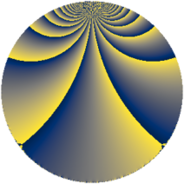# Properties

 Label 1344.4.caLevel $1344$ Weight $4$ Character orbit 1344.ca Rep. character $\chi_{1344}(271,\cdot)$ Character field $\Q(\zeta_{12})$ Dimension $384$ Sturm bound $1024$

# Related objects

## Defining parameters

 Level: $$N$$ $$=$$ $$1344 = 2^{6} \cdot 3 \cdot 7$$ Weight: $$k$$ $$=$$ $$4$$ Character orbit: $$[\chi]$$ $$=$$ 1344.ca (of order $$12$$ and degree $$4$$) Character conductor: $$\operatorname{cond}(\chi)$$ $$=$$ $$112$$ Character field: $$\Q(\zeta_{12})$$ Sturm bound: $$1024$$

## Dimensions

The following table gives the dimensions of various subspaces of $$M_{4}(1344, [\chi])$$.

Total New Old
Modular forms 3136 384 2752
Cusp forms 3008 384 2624
Eisenstein series 128 0 128

## Trace form

 $$384q + O(q^{10})$$ $$384q + 40q^{11} - 656q^{23} - 800q^{29} - 456q^{35} + 16q^{37} + 1616q^{43} - 752q^{53} - 4128q^{59} - 2256q^{67} + 896q^{71} + 15552q^{81} + 3496q^{91} + 720q^{99} + O(q^{100})$$

## Decomposition of $$S_{4}^{\mathrm{new}}(1344, [\chi])$$ into newform subspaces

The newforms in this space have not yet been added to the LMFDB.

## Decomposition of $$S_{4}^{\mathrm{old}}(1344, [\chi])$$ into lower level spaces

$$S_{4}^{\mathrm{old}}(1344, [\chi]) \cong$$ $$S_{4}^{\mathrm{new}}(112, [\chi])$$$$^{\oplus 6}$$$$\oplus$$$$S_{4}^{\mathrm{new}}(336, [\chi])$$$$^{\oplus 3}$$$$\oplus$$$$S_{4}^{\mathrm{new}}(448, [\chi])$$$$^{\oplus 2}$$